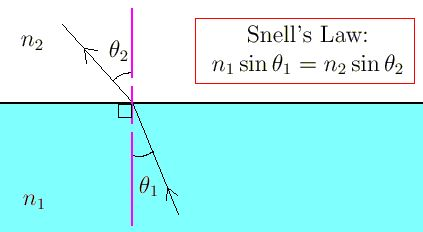# Direction : The question has a paragraph followed by two statements, Statement-1 and Statement-2. Of the given four alternatives after the statements, choose the one that describes the statements.A thin air film is formed by putting the convex surface of a plane-convex lens over a plane glass plate. With monochromatic light, this film gives an interference pattern due to light reflected from the top (convex) surface and the bottom (glass plate) surface of the film.Statement - 1: When light reflects from the air- glass plate interface, the reflected wave suffers a phase change of .Statement - 2: The centre of the interference pattern is dark. Option 1) Statement-1 is true, Statement-2 is false. Option 2) Statement-1 is true , Statement-2 is true, Statement-2 is the correct explanation of Statement-1. Option 3) Statement-1 is true, Statement-2 is true, Statement-2 is not the correct explanation of Statement-1. Option 4) Statement-1 is false, Statement-2 is true.

P Prateek Shrivastava

As we learnt in

Relation between angle of incidence and angle of refaction -

- whereinrefractive index of medium of incidence.

refractive index of medium where rays is refracted.

angle of incidence.

angle of refraction.

Thin lens formula -

- wherein

are object and image distance from lens.

S1= When light reflects from denser (glass) a phase drift of  is generated.

S2= Centre maximum or minima depends on thickness of the lens.

Correct option is 3.

Option 1)

Statement-1 is true, Statement-2 is false.

This is an incorrect option.

Option 2)

Statement-1 is true , Statement-2 is true, Statement-2 is the correct explanation of Statement-1.

This is an incorrect option.

Option 3)

Statement-1 is true, Statement-2 is true, Statement-2 is not the correct explanation of Statement-1.

This is the correct option.

Option 4)

Statement-1 is false, Statement-2 is true.

This is an incorrect option.

Exams
Articles
Questions